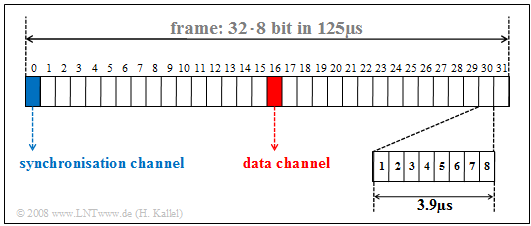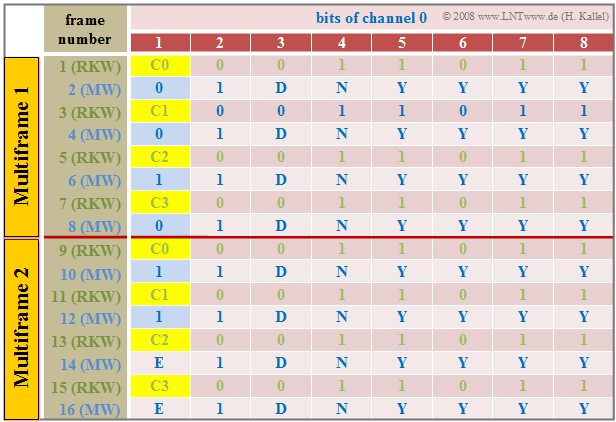# ISDN Primary Multiplex Connection

$\text{Preliminary remark:}$  Again,  we would like to point out that the content of this chapter no longer fully reflects the current state of the art  $($2018$)$.  Therefore,  consider the following text as a historical treatise,  even if parts of it are still relevant in practice today.

## General description

First of all,  it should be explained why an ISDN rate interface is  $($or rather was$)$  needed.  This was only offered as a   system connection   $($"point-to-point"$)$. This means that only one device could be connected to the network termination:  One speaks of a  "telecommunications system",  abbreviated to  "TC system"  in the following.

There were many reasons for using a system connection:

1. Companies,  government agencies or hospitals often need a central number and a block of extension numbers.
2. Most often,  the extension number of the central office is  "0".
3. The central call number has  $3$  to  $5$  digits,  an extension number thereafter has  "2"  to  "5"  digits.  This allows direct dialing of a call partner from the outside.
4. Telephone calls between employees  – i.e.,  an internal connection –  should be free of charge.

$\text{Example 1:}$  Let's consider a company in Munich whose head office can be reached from outside via  "089/4711 - 0"  and internally with  "0".  Employee  $X$  can be reached from outside at a charge by dialing extension  "089/4711 - 432"  and internally without charge by dialing  "432".

Larger companies usually work with a   primary rate interface   $\rm (PRI)$,  to which the telecommunications or data processing equipment is connected by a four-wire line.  The primary rate interface according to the adjacent diagram offers:

• $30$  full-duplex basic channels with  $\text{64 kbit/s}$  each,
• one signaling channel  $($"data channel"$)$  with  $\text{64 kbit/s}$,
• one synchronization channel  (also with  $\text{64 kbit/s})$,  and accordingly
• gross data rate  of  $32 · 64 \hspace{0.15cm}\underline{ = 2048 \ \rm kbit/s}$.

It follows some general information about the primary rate interface:

1. The  $30$  user channels are implemented with the  "PCM-30"  multiplex system.  In contrast to the basic rate interface,  only a point-to-point connection is possible here.  This means that a second system cannot be connected to the same line as with a bus.
2. The telecommunications system is connected to the local exchange via the network termination equipment  $($"Network Termination for Primary Rate Multiplex Access"   ⇒   "$\rm NTPM$"$)$.  This connection is four-wire   ⇒   both transmission directions are separated.  Thus,  no direction separation procedures  $($fork circuit,  echo cancellation,  etc.$)$  are required in the NTPM and in the local exchange.
3. The  "reference point"  $\rm U$  between the network termination and the local exchange is designated  $\rm U_{K2}$ in the case of a primary multiplex connection if a copper cable  $\rm (K)$  is used; the  $\rm (2)$  stands for the transmission rate of  $\text{2 Mbit/s}$.  In the case of a fiber optic connection,  this point is called  $\rm U_{G2}$.
4. Accordingly,  the connection between the network termination and the TC system is generally referred to as the  "$\rm S_{2M}$"  interface.  Technically, however,  there is not much difference between the  "$\rm U_{K2}$"  and the  "$\rm S_{2M}$"  interfaces.

## Frame structure of S2M and UK2 interface

The  $\rm S_{2M}$ interface represents the connection between the telecommunications system and the network termination  $\rm (NTPM)$,  which is implemented with two copper pairs.  Since only point-to-point operation is possible here,  the  $\rm S_{2M}$ interface is not designed as a bus like the  $\rm S_{0}$ interface in the basic connection,  and therefore no collision detection method is required here.Frame structure of the  $\rm S_{2M}$ interface

The graphic shows the frame structure of the  $\rm S_{2M}$ interface. It can be seen:

• In time division multiplex,  a TDMA frame is transmitted every  $125\ \rm µs$.  However,  each of the  $32$  channels occupies the TDMA frame only for the duration of  $125\ \rm µs/32 = 3.906 \ \rm µs$.
• Eight bits are transmitted per channel and TDMA frame; the bit duration is
$$T_\text{B} = 3.906 \ \rm µs/8 = 0.488 \ \rm µs.$$
• The reciprocal of this is the gross data rate
$$R_\text{B} \hspace{0.15cm}\underline{= 2.048 \ \rm Mbit/s}.$$
• The channels  1  to  15  and  17  to  31  represent the bearer  ($\rm B)$  channels,  all of which are operated independently of each other at  $64 \ \rm kbit/s$.
• The data channel  16  $($marked red in the graph$)$  provides control of these user channels and the entire telephone system.
• The synchronization channel  0  $($marked in blue$)$  is used for frame detection in the case of odd frames  $($with number  $1, 3, 5,$ ...$)$,  while the even frames  ($2, 4, 6,$ ...$)$  are used for maintenance purposes and for error handling.  Both are done with the help of the  $\rm CRC4$  method,  which is described in more detail in the next section.

The  $\rm U_{K2}$ interface has exactly the same properties as the  $\rm S_{2M}$ interface and thus also has the same frame structure.

## Frame synchronization

Synchronization is implemented in the  channel  "0"  of a frame in the primary rate interface.  The table shows the respective frame assignment of this synchronization channel  for one cycle of the CRC4 method.  The  "Cyclic Redundancy Check"  $\rm (CRC4)$  is used for this purpose,  which can be illustrated briefly as follows:Frame assignment of the synchronization channel  "0"
1.   Channel  "0"  of each odd time frame  $($number 1, 3, ... , 15$)$  includes the  "frame password" $($German:  "Rahmenkennwort"   ⇒   "RKW"$)$,  while each even frame  $($number 2, 4, ... , 16$)$  of this channel contains the  "message word".
2.   Based on the frame password with the fixed bit pattern  "$\rm X001\hspace{0.08cm} 1011$",  the synchronization between the transmission and the reception direction is established.  The first bit  $\rm X ∈ {0, 1}$  is determined by the CRC4 method.
3.   The message word is  "$\rm X1DN\hspace{0.08cm} YYYY$".  Error messages  are signaled via the  $\rm D$ bit and the  $\rm N$ bit.  The four  $\rm Y$ bits are reserved for service functions.  The  $\rm X$ bit is again obtained by the CRC4 method.
4.   The CRC4 method requires  $16$  $\rm X$ bits   ⇒   $16$  consecutive pulse frames,  which are divided into two multiple frames.  The length of a multiple frame is therefore  $8 · 256 = 2048$  bits and the time duration is  $8 · 0.125 = 1$  millisecond.
5.   The CRC4 checksum is formed as a sequence of four bits  $(\rm C0$, ... , $\rm C3)$  in each multiple frame and provides the first bit  $\rm (X)$  for each of four consecutive frame identifiers.

$\text{Example 2:}$  The procedure of the CRC4 method shall be explained by an example, where for the generator polynomial is assumed:

$$D^4 + D + 1$$

In the binary representation this is:  "$10011$".  The graph shows the extraction of the CRC4 checksum  (left)  and its evaluation at the receiver  (right). You can see:

• The CRC4 checksum at the transmitter-side results as the remainder of the division of a data block with a total of twelve bits  $($eight useful bits,  here  "$1000\hspace{0.05cm} 1100$",  to which  "$0000$" is appended$)$  by the generator polynomial  "$10011$".
• In polynomial notation, the remainder of the division
$$(D^{11} + D^7 + D^6 ) : ( D^4 + D + 1)$$
results in  $R(D) = D^3 + 1$    ⇒   binary "$1001$".
• The division is realized by a  "modulo-2 addition"  $($bitwise XOR operation$)$.  In the example,  the division yields the remainder  "$1001$".
• These bits  $\rm C0$, ... , $\rm C3$  of the CRC checksum are transmitted to the receiver in different frames of the synchronization channel  $($see frame assignment in the above graphic$)$.
• After the receiver has received these twelve bits  $($data block and CRC4 checksum$)$,  it also divides this 12-digit binary word by the generator polynomial.  In the example,  this division  "$1000\hspace{0.05cm} 1100\hspace{0.05cm} 1001$"  divided by  "$10011$"  gives the remainder zero.  This result indicates that no transmission errors have occurred.
• If the division remainder is not zero,  the result indicates a transmission error.  In this case,  the data must be requested again from the transmitter.

## Telecommunications aspects

With the ISDN primary rate interface,  the so-called   HDB3 line code   $($High Density Bipolar 3ary$)$  is used on the  $\rm S_{2M}$ interface and also on the  $\rm U_{K2}$ interface.  Compared to the modified AMI code on the  $\rm S_{0}$ interface of the base connection

• the occurrence of long zero sequences is avoided,  and thus
• providing the receiver a more reliable clock recovery and synchronization.

HDB3 line coding works as follows:

1. As in the AMI code,  each binary  "0"  is assigned the signal level  $\rm 0\hspace{0.09cm} V$,  while the binary "1" is alternately represented by the values  $+s_0$  and  $–s_0$.
2. If four consecutive  "0"  bits occur in the AMI encoded signal,  they are replaced by a sequence of four other bits that violate the AMI coding rule.
3. If,  as in the above figure,  the number of  "ones"  is even or zero and the last pulse before these four bits is negative,  "0 0 0 0"  is replaced by the sequence  "+ 0 0 +".  On the other hand,  if the last pulse before these four bits were positive,  "0 0 0 0" would be replaced by "– 0 0 –".
4. If there were an odd number of  "ones"  before this  "0 0 0 0"  block,  on the other hand,  "0 0 0 +"  $($if last pulse positive$)$  or  "0 0 0 –"  $($if last pulse negative$)$  would be selected as replacements.  The DC freedom is maintained by these measures.
5. In all four cases,  the decoder can detect the violation of the AMI rule and replace this block again with  "0 0 0 0".